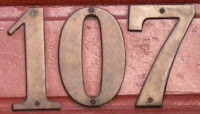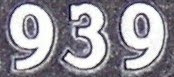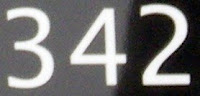## Friday, December 23, 2011

### 2012

2012 = 22 x 503.

2012, 2013, 2014, and 2015 are each the product of three prime numbers.

2012! has a square number of digits (5776 = 762).

20122 = 4048144 and 4418404 = 21022.

2012 and its square (4048144) use only the digits 0, 1, 2, 4, and 8.2012 is a year in which there are five Wednesdays in the month of February.

2012 is a blue moon year.

The end of the "longest period" (Long Count) Mayan calendar known to exist is said to be December 21, 2012.

2012 is the 100th anniversary of the sinking of the RMS Titanic. It is also the centennial of the birth of Alan Turing.

## Thursday, December 22, 2011

### 524

524 = 22 x 131.

524 is a divisor of 535 - 1.

6 x 7 x 9 x 524 x 831 = 164,597,832 uses each of the digits from 1 to 9 once on both sides of the equality.

524 is 201102 (palindromic) in base 3.The USS Pickerel (USN-524) was a submarine commissioned in 1949.

## Wednesday, December 21, 2011

### 1011

1011 = 3 x 337.

1011 is the smallest number without trailing zeroes such that the reversal of its cube is the cube of a different number (1101).

1011 has a square that is formed by inserting three 2s into it: 10112 = 1022121.

1011 is 323 (palindromic) in base 18. 1011 has a representation ending in 3 in base 4, 6, 7, 8, 9, 12, 14, and 18.The Nokia 1011 was the first mass-produced GSM phone.

## Tuesday, December 20, 2011

### 107

107 is a prime number.

107 is 1101011 in base 2 (binary).

107 and 109 form a twin prime pair.

2107 - 1 is a Mersenne prime.

107 is the smallest three-digit prime that remains a prime when 2 is added to any one of its digits: 307, 127, and 109 are all primes.

107 is the maximum number of steps that a four-state Turing machine can make on an initially blank tape before it halts.107 Camilla is one of the largest main-belt asteroids.

Source: Prime Curios!

## Monday, December 19, 2011

### 383

383 is a prime number. It is a sexy prime because 383 + 6 = 389 is also prime.

383 is the only known multidigit palindromic Woodall prime.

383 is the sum of the first three three-digit palindromic primes: 383 = 101 + 131 + 151.

383 is the smallest prime that can be represented by the sum of a prime and its reversal: 383 = 241 + 142.

383 is a divisor of the Mersenne number 2191 - 1.The Chevy 383 stroker engine uses an altered crankshaft to increase the stroke of the pistons.

## Friday, December 16, 2011

### 967

967 is a prime number. 967 in reverse is the prime 769.

967 is a divisor of 977 - 1.

967 is the number of six-digit triangular numbers.

967 is 595 in base 13.967 millimeters is the record for annual rainfall in Melbourne, Australia, set in 1916.

## Thursday, December 15, 2011

### 743

743 is a prime number. It is a Sophie Germain prime because 2 x 743 + 1 = 1487 is a prime.

743 is the number of independent sets of the graph of the tesseract (four-dimensional cube).

6487 + 1399684 = 75582723.

743 is 616 in base 11 and 212 in base 19.The sun's mass is about 743 times the combined mass of all the planets in the solar system.

## Wednesday, December 14, 2011

### 1352

1352 = 23 x 132.

1352 has two representations as a sum of two squares: 1352 = 142 + 342 = 262 + 262.

1352 is a divisor of 994 - 1.

1352 is 20402 in base 5 and 242 in base 25. It is 800 in base 13 and 200 in base 26.NGC 1352 is a barred spiral galaxy in the constellation Eridanus.

## Tuesday, December 13, 2011

### 197

197 is a prime number.

197 is the sum of the first 12 primes: 197 = 2 + 3 + 5 + 7 + 11 + 13 + 17 + 19 + 23 + 29 + 31 + 37. It is also the smallest prime that is the sum of seven consecutive primes: 197 = 17 + 19 + 23 + 29 + 31 + 37 + 41.

197 is the sum of the digits of all two-digit primes.

197 is the sum of the squares of all composite digits: 197 = 42 + 62 + 82 + 92.

197 has a representation as a sum of two squares: 197 = 12 + 142.

197 and 199 form a twin prime pair.

197 is the hypotenuse of a primitive Pythagorean triple: 1972 = 282 + 1952.The chemical element gold has one stable isotope, which has a total of 197 neutrons and protons in its nucleus.

## Monday, December 12, 2011

### 647

647 is a prime number.

647 is a divisor of 4317 - 1.

647 is the smallest prime that can be written as 2a2 - 1 and as 3b3 - 1.

647 is 343 in base 14.

647 is the sum of five consecutive primes: 647 = 113 + 127 + 131 + 137 + 139.Tremont 647 is a restaurant in Boston.

## Friday, December 9, 2011

### 286

286 = 2 x 11 x 13.

286 is the 11th tetrahedral number.

286 is the number of rooted trees with 9 vertices.

286 is 2121 in base 5 and 141 in base 15.286 is the shorthand for the Intel 80286 microprocessor chip.

## Thursday, December 8, 2011

### 360

360 = 23 x 32 x 5.

360 has a representation as a sum of two squares: 360 = 62 + 182.

360 is the largest number out of two (the other being 36) that have the same number of letters in its Roman numeral representation (CCCLX) as its double (DCCXX), triple (MLXXX), quadruple (MCDXL), quintuple (MDCCC), sextuple (MMCLX), and septuple (MMDXX).

360 is the smallest number divisible by every natural number from 1 to 10 except 7.

One of the divisors of 360 is 72, which is the number of prime numbers less than 360.

360 is the sum to two twin primes: 360 = 179 + 181.360 is the number of degrees in a circle.

## Wednesday, December 7, 2011

### 939

939 = 3 x 313.

939 is a divisor of 254 - 1.

939 has a cube root whose decimal part starts with the digits 1 to 9 in some order: 9.792386145. . .

939 is 32223 in base 4 and 12224 in base 5.

939 is a number n such that n - 2 and n + 2 are consecutive primes: 937 and 941.The Berklee College of Music's Cafe 939 is a student-run music venue and coffeehouse.

## Tuesday, December 6, 2011

### 175

175 = 52 x 7.

175 is a divisor of 762 - 1.

175 = 11 + 72 + 53.

175 is the smallest number n such that n6 + 6 is prime.Emory University was 175 years old in the year 2o11.

## Monday, December 5, 2011

### 831

831 = 3 x 277.

6 x 7 x 9 x 524 x 831 = 164,597,832 (using each of the nine digits 1 to 9 on both sides of the equality).

831 is a divisor of 3512 - 1.

831 is 11311 in base 5 and 696 in base 11.

831 is the sum of 12 positive fifth powers: 831 = 35 + 35 + 35 + 25 + 25 + 25 + 15 + 15 + 15 + 15 + 15 + 15.831 stands for "I love you": eight letters, three words, one meaning.

Source: Numeropedia

## Friday, December 2, 2011

### 342

342 = 2 x 32 x 19.

342 is 666 in base 7. It is the smallest number that can be represented by 666 in any base. 342 is also 2332 in base 5.

342 is the number of inequivalent binary linear codes of length 8.IC 342 (also known as Caldwell 5) is a spiral galaxy in the constellation Camelopardalis.

## Thursday, December 1, 2011

### 727

727 is a prime number.

727 is the smallest prime of the form n3 - 2.

727 = 1! + (1 + 2)! + (1 + 2 + 3)!

727 is the smallest odd prime that can be represented as the sum of a cube and its reversal: 727 = 83 + 215 = 512 + 215.

727 is the first prime whose square (528529) can be represented as the concatenation of two consecutive numbers.The Boeing 727 was the first three-engine jet built for commercial service.